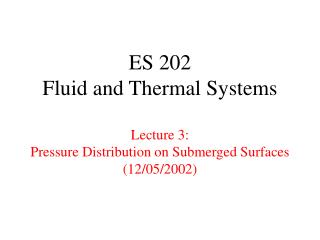DownloadDownload PresentationES 202 Fluid and Thermal Systems Lecture 3: Pressure Distribution on Submerged Surfaces (12/05/2002)

ES 202 Fluid and Thermal Systems Lecture 3: Pressure Distribution on Submerged Surfaces (12/05/2002)

Télécharger la présentationES 202 Fluid and Thermal Systems Lecture 3: Pressure Distribution on Submerged Surfaces (12/05/2002)

- - - - - - - - - - - - - - - - - - - - - - - - - - - E N D - - - - - - - - - - - - - - - - - - - - - - - - - - -
Presentation Transcript

1. ES 202Fluid and Thermal SystemsLecture 3:Pressure Distribution on Submerged Surfaces(12/05/2002)

2. Assignments • Reading: • Cengel & Turner Section 10-4 and 10-5 • Homework: • 10-11, 10-19, 10-37 in Cengel & Turner • Definition of specific gravity and gage pressure

3. Road Map of Lecture 3 • Announcements • Quiz on Lecture 2 • Examples and exercises • Conceptual exercises • Pressure distribution at various depths and arbitrary shapes • Pressure distribution in multiple layers of different fluids • Application of manometer • Force and moment on vertically submerged surface • Concept of resultant force and center of pressure

4. Announcements • First homework set due by 5 pm next Monday at my office • Problem 10-37 is moved to Lecture 4 (next Monday) • Comments on write-up for Lab #1 • Review body force and surface force in ES 201 For Section 06 only: • Location of Lab 1 at B105, not the lecture classroom

5. Forces acted on a system can generally be classified as: body force surface force Surface force can further be classified as: shear force normal force All about pressure: Pressure is a vector/scalar. Pressure is a body/surface force. Pressure acts in the shear/normal direction. Pressure is a compressive/expansive force. Quiz on Lecture 2

6. Pressure is the same/different in different directions at any single point in a fluid. Pressure variation in a static fluid: Variation in horizontal direction P = constant Variation in vertical direction d P / d y = - r g Give a physical meaning/interpretation to the above equation It is a balance between surface force and body force in a static fluid. Quiz on Lecture 2 (Cont’d)

7. Conceptual Exercises • Pressure at various depths and arbitrary shape • Compare the pressure at various locations as indicated • What does the linear plot represent in the figure? • Does the figure show all the pressure forces present in the system? • If necessary, what modification will you make to include all the pressure forces in the system? • Pressure in multiple layers of different fluids • Draw the gage pressure distribution as a function of fluid depth • Discussion of pressure at the interface between fluids

8. Manometer • Definition: Device used to measure small to moderate pressure difference based on the difference in height of fluid columns • Manometer problem • What are the pressure values at the selected locations? • “Swim” through the fluid as you track down the pressure distribution

9. Vertically Submerged Surface • Fish tank problem • Estimate the total weight of water contained (intuition) • What is the pressure at the bottom of the tank? • Sketch the pressure distribution on the side wall? • Find the total force on the side wall • Find the total moment on the side wall about Point A • Find the center of pressure (take a first guess at its approximate location)